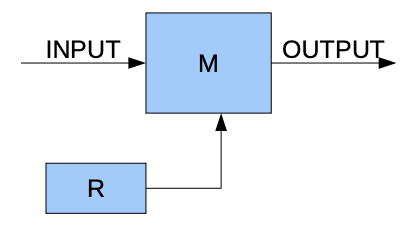시간 제한메모리 제한제출정답맞힌 사람정답 비율
1 초 64 MB67241425.926%

## 문제

There is a rumor that the Scientific Committee is using a special device for encrypting their communication. If you can crack the encryption you could listen to the problems they have prepared and score many points. Last night you got lucky: one of the members forgot their device in a bar. You opened it and looked at the general design:All the operations use 8 bits. XOR is the bitwise exclusive or function (the ^ operator in C, xor operator in Pascal).

R is a sequence of pseudorandom numbers defined as follows:

• R, R and R have secret values only known by the Scientific Committee.
• For n = 3,4,… let R[n] = R[n-2] XOR R[n-3].

Furthermore, we have a function M, M:[0..255] →[0..255]. In fact, M is a bijection, i.e. when x≠y it must also be that M[x]≠M[y].

The device used by the Scientific Committee takes one number at a time, and outputs it encrypted.

After using the device N times, the next number, INPUT is encoded as follows:

• OUTPUT = M(INPUT XOR R[N])

Even though you understand how the encryption device works, you do not know the secret values R, R, and R. Also, you do not know M, so you cannot decode the communication. What you can do instead is play with the device you found. You can give it input numbers and observe the outputs.

Your task is to find out all the secret values: R, R, R, M, M, .., M with less than 320 queries (input numbers).

## 인터랙션

This is an interactive program. To play with the encryption module simply write a number between 0 and 255 to the standard output. You can then read from the standard input the output of the encryption device, also an integer between 0 and 255. When you know the secret values output one line containing the string SOLUTION and after that output 259 lines: R, R, R, M, M, …, M, one value per line.

## 제한

• A correct solution receives points only if the number of queries is less than 320.
• In all tests the secret keys R, R, R, M, M, …, M are random.
• 0 ≤ INPUT, OUTPUT, R, M ≤ 255

## 예제

Let's assume that R = 0, R = 1, R = 3 and that M[i] = (i + 1) MODULO 256. From here we deduce R = 1.

10 11 M[10 XOR 0] = M = 11
10 12 M[10 XOR 1] = M = 12
11 9 M[11 XOR 3] = M = 9
12 14 M[12 XOR 1] = M = 14
...
SOLUTION
0
1
3
1
2
3
4

254
255
0
When you find out the secret values you output them.

## 채점 및 기타 정보

• 예제는 채점하지 않는다.